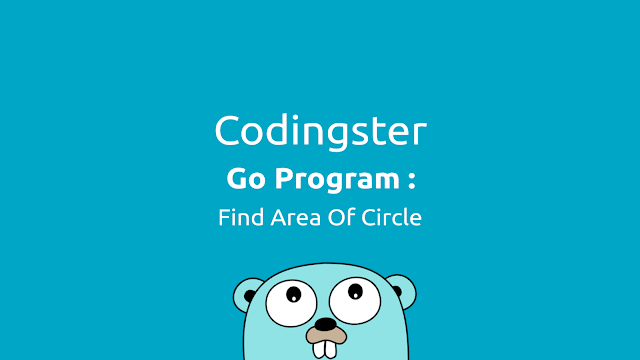-->

## Social Items

Go Program To Find Area Of Circle - This Go program to calculate an area of a circle, The program takes the radius of the circle as input, calculates the area of the circle and outputs it on the screen.

### Source Code :

``````package main

import "fmt"

func main() {
const phi float64 = 3.14

fmt.Println("Area of circle is: ", area)
}
``````

### Compile & Run :

Here's how to compile source code manually:
``````
\$ go build areaofcircle.go
\$ ./areaofcircle
``````
Run without compile:
``````
\$ go run areaofcircle.go
``````

### Output Of Program :The result of Golang program to find area of circle## Go Program To Find Area Of Circle (Golang)

Go Program To Find Area Of Circle - This Go program to calculate an area of a circle, The program takes the radius of the circle as input, calculates the area of the circle and outputs it on the screen.

### Source Code :

``````package main

import "fmt"

func main() {
const phi float64 = 3.14

fmt.Println("Area of circle is: ", area)
}
``````

### Compile & Run :

Here's how to compile source code manually:
``````
\$ go build areaofcircle.go
\$ ./areaofcircle
``````
Run without compile:
``````
\$ go run areaofcircle.go
``````

### Output Of Program :The result of Golang program to find area of circle